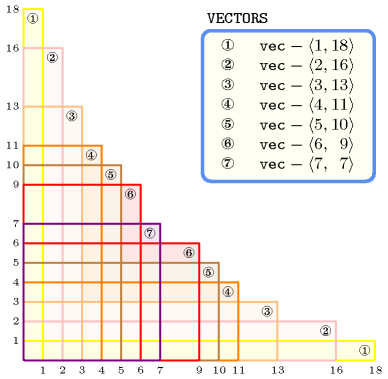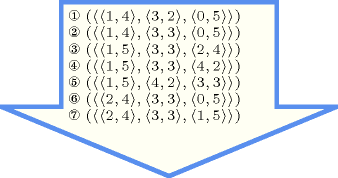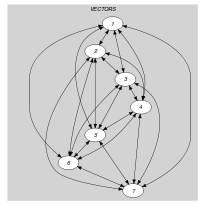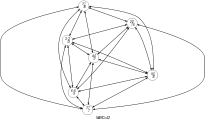## 5.10. all_incomparable

Origin

Inspired by incomparable rectangles.

Constraint

$\mathrm{𝚊𝚕𝚕}_\mathrm{𝚒𝚗𝚌𝚘𝚖𝚙𝚊𝚛𝚊𝚋𝚕𝚎}\left(\mathrm{𝚅𝙴𝙲𝚃𝙾𝚁𝚂}\right)$

Synonym

$\mathrm{𝚊𝚕𝚕}_\mathrm{𝚒𝚗𝚌𝚘𝚖𝚙𝚊𝚛𝚊𝚋𝚕𝚎𝚜}$.

Type
 $\mathrm{𝚅𝙴𝙲𝚃𝙾𝚁}$ $\mathrm{𝚌𝚘𝚕𝚕𝚎𝚌𝚝𝚒𝚘𝚗}\left(\mathrm{𝚟𝚊𝚛}-\mathrm{𝚍𝚟𝚊𝚛}\right)$
Argument
 $\mathrm{𝚅𝙴𝙲𝚃𝙾𝚁𝚂}$ $\mathrm{𝚌𝚘𝚕𝚕𝚎𝚌𝚝𝚒𝚘𝚗}\left(\mathrm{𝚟𝚎𝚌}-\mathrm{𝚅𝙴𝙲𝚃𝙾𝚁}\right)$
Restrictions
 $\mathrm{𝚛𝚎𝚚𝚞𝚒𝚛𝚎𝚍}$$\left(\mathrm{𝚅𝙴𝙲𝚃𝙾𝚁},\mathrm{𝚟𝚊𝚛}\right)$ $|\mathrm{𝚅𝙴𝙲𝚃𝙾𝚁}|\ge 1$ $\mathrm{𝚛𝚎𝚚𝚞𝚒𝚛𝚎𝚍}$$\left(\mathrm{𝚅𝙴𝙲𝚃𝙾𝚁𝚂},\mathrm{𝚟𝚎𝚌}\right)$ $|\mathrm{𝚅𝙴𝙲𝚃𝙾𝚁𝚂}|\ge 1$ $\mathrm{𝚜𝚊𝚖𝚎}_\mathrm{𝚜𝚒𝚣𝚎}$$\left(\mathrm{𝚅𝙴𝙲𝚃𝙾𝚁𝚂},\mathrm{𝚟𝚎𝚌}\right)$
Purpose

Enforce for each pair of distinct vectors of the $\mathrm{𝚅𝙴𝙲𝚃𝙾𝚁𝚂}$ collection the fact that they are incomparable. Two vectors $\mathrm{𝚅𝙴𝙲𝚃𝙾𝚁}\mathtt{1}$ and $\mathrm{𝚅𝙴𝙲𝚃𝙾𝚁}\mathtt{2}$ are incomparable if and only, when the components of both vectors are ordered, and respectively denoted by $\mathrm{𝚂𝚅𝙴𝙲𝚃𝙾𝚁}\mathtt{1}$ and $\mathrm{𝚂𝚅𝙴𝙲𝚃𝙾𝚁}\mathtt{2}$, we neither have $\mathrm{𝚂𝚅𝙴𝙲𝚃𝙾𝚁}\mathtt{1}\left[i\right].\mathrm{𝚟𝚊𝚛}\le \mathrm{𝚂𝚅𝙴𝙲𝚃𝙾𝚁}\mathtt{2}\left[i\right].\mathrm{𝚟𝚊𝚛}$ (for all $i\in \left[1,|\mathrm{𝚂𝚅𝙴𝙲𝚃𝙾𝚁}\mathtt{1}|\right]$) nor have $\mathrm{𝚂𝚅𝙴𝙲𝚃𝙾𝚁}\mathtt{2}\left[i\right].\mathrm{𝚟𝚊𝚛}\le \mathrm{𝚂𝚅𝙴𝙲𝚃𝙾𝚁}\mathtt{1}\left[i\right].\mathrm{𝚟𝚊𝚛}$ (for all $i\in \left[1,|\mathrm{𝚂𝚅𝙴𝙲𝚃𝙾𝚁}\mathtt{1}|\right]$).

Example
$\left(\begin{array}{c}〈\begin{array}{c}\mathrm{𝚟𝚎𝚌}-〈1,18〉,\hfill \\ \mathrm{𝚟𝚎𝚌}-〈2,16〉,\hfill \\ \mathrm{𝚟𝚎𝚌}-〈3,13〉,\hfill \\ \mathrm{𝚟𝚎𝚌}-〈4,11〉,\hfill \\ \mathrm{𝚟𝚎𝚌}-〈5,10〉,\hfill \\ \mathrm{𝚟𝚎𝚌}-〈6,9〉,\hfill \\ \mathrm{𝚟𝚎𝚌}-〈7,7〉\hfill \end{array}〉\hfill \end{array}\right)$

The $\mathrm{𝚊𝚕𝚕}_\mathrm{𝚒𝚗𝚌𝚘𝚖𝚙𝚊𝚛𝚊𝚋𝚕𝚎}$ constraint holds since all distinct pairs of vectors are incomparable as illustrated by Figure 5.10.1.

##### Figure 5.10.1. Illustrating the incomparability of vectors $〈1,18〉$, $〈2,16〉$, $〈3,13〉$, $〈4,11〉$, $〈5,10〉$, $〈6,9〉$, $〈7,7〉$: first to each vector we associate a rectangle whose sizes are the components of the vector; second no matter whether we rotate a rectangle from ${90}^{\circ }$ or not, one rectangle can not be included in another rectangle.All solutions

Figure 5.10.2 gives all solutions to the following non ground instance of the $\mathrm{𝚊𝚕𝚕}_\mathrm{𝚒𝚗𝚌𝚘𝚖𝚙𝚊𝚛𝚊𝚋𝚕𝚎}$ constraint: ${U}_{1}\in \left[1,2\right]$, ${V}_{1}\in \left[0,5\right]$, ${U}_{2}\in \left[3,5\right]$, ${V}_{2}\in \left[2,3\right]$, ${U}_{3}\in \left[0,6\right]$, ${V}_{3}\in \left[2,5\right]$, $\mathrm{𝚊𝚕𝚕}_\mathrm{𝚒𝚗𝚌𝚘𝚖𝚙𝚊𝚛𝚊𝚋𝚕𝚎}$$\left(〈〈{U}_{1},{V}_{1}〉,〈{U}_{2},{V}_{2}〉,〈{U}_{3},{V}_{3}〉〉\right)$.

##### Figure 5.10.2. All solutions corresponding to the non ground example of the $\mathrm{𝚊𝚕𝚕}_\mathrm{𝚒𝚗𝚌𝚘𝚖𝚙𝚊𝚛𝚊𝚋𝚕𝚎}$ constraint of the All solutions slotTypical
 $|\mathrm{𝚅𝙴𝙲𝚃𝙾𝚁}|>1$ $|\mathrm{𝚅𝙴𝙲𝚃𝙾𝚁𝚂}|>1$ $|\mathrm{𝚅𝙴𝙲𝚃𝙾𝚁𝚂}|>|\mathrm{𝚅𝙴𝙲𝚃𝙾𝚁}|$
Symmetry

Items of $\mathrm{𝚅𝙴𝙲𝚃𝙾𝚁𝚂}$ are permutable.

Arg. properties

Contractible wrt. $\mathrm{𝚅𝙴𝙲𝚃𝙾𝚁𝚂}$.

Keywords
Cond. implications

$•$ $\mathrm{𝚊𝚕𝚕}_\mathrm{𝚒𝚗𝚌𝚘𝚖𝚙𝚊𝚛𝚊𝚋𝚕𝚎}\left(\mathrm{𝚅𝙴𝙲𝚃𝙾𝚁𝚂}\right)$

with  $|\mathrm{𝚅𝙴𝙲𝚃𝙾𝚁}|=2$

implies $𝚔_\mathrm{𝚍𝚒𝚜𝚓𝚘𝚒𝚗𝚝}$$\left(\mathrm{𝚂𝙴𝚃𝚂}:\mathrm{𝚅𝙴𝙲𝚃𝙾𝚁𝚂}\right)$.

$•$ $\mathrm{𝚊𝚕𝚕}_\mathrm{𝚒𝚗𝚌𝚘𝚖𝚙𝚊𝚛𝚊𝚋𝚕𝚎}\left(\mathrm{𝚅𝙴𝙲𝚃𝙾𝚁𝚂}\right)$

with  $|\mathrm{𝚅𝙴𝙲𝚃𝙾𝚁}|=2$

implies $\mathrm{𝚝𝚠𝚒𝚗}$$\left(\mathrm{𝙿𝙰𝙸𝚁𝚂}:\mathrm{𝚅𝙴𝙲𝚃𝙾𝚁𝚂}\right)$.

Arc input(s)

$\mathrm{𝚅𝙴𝙲𝚃𝙾𝚁𝚂}$

Arc generator
$\mathrm{𝐶𝐿𝐼𝑄𝑈𝐸}$$\left(\ne \right)↦\mathrm{𝚌𝚘𝚕𝚕𝚎𝚌𝚝𝚒𝚘𝚗}\left(\mathrm{𝚟𝚎𝚌𝚝𝚘𝚛𝚜}\mathtt{1},\mathrm{𝚟𝚎𝚌𝚝𝚘𝚛𝚜}\mathtt{2}\right)$

Arc arity
Arc constraint(s)
$\mathrm{𝚒𝚗𝚌𝚘𝚖𝚙𝚊𝚛𝚊𝚋𝚕𝚎}$$\left(\mathrm{𝚟𝚎𝚌𝚝𝚘𝚛𝚜}\mathtt{1}.\mathrm{𝚟𝚎𝚌},\mathrm{𝚟𝚎𝚌𝚝𝚘𝚛𝚜}\mathtt{2}.\mathrm{𝚟𝚎𝚌}\right)$
Graph property(ies)
$\mathrm{𝐍𝐀𝐑𝐂}$$=|\mathrm{𝚅𝙴𝙲𝚃𝙾𝚁𝚂}|*|\mathrm{𝚅𝙴𝙲𝚃𝙾𝚁𝚂}|-|\mathrm{𝚅𝙴𝙲𝚃𝙾𝚁𝚂}|$

Graph class
 $•$$\mathrm{𝙽𝙾}_\mathrm{𝙻𝙾𝙾𝙿}$ $•$$\mathrm{𝚂𝚈𝙼𝙼𝙴𝚃𝚁𝙸𝙲}$

Graph model

The Arc constraint(s) slot uses the $\mathrm{𝚒𝚗𝚌𝚘𝚖𝚙𝚊𝚛𝚊𝚋𝚕𝚎}$ constraint defined in this catalogue.

Parts (A) and (B) of Figure 5.10.3 respectively show the initial and final graph associated with the Example slot. Since we use the $\mathrm{𝐍𝐀𝐑𝐂}$ graph property, the arcs of the final graph are stressed in bold. The previous constraint holds since exactly $3·\left(3-1\right)=6$ arc constraints hold.

##### Figure 5.10.3. Initial and final graph of the $\mathrm{𝚊𝚕𝚕}_\mathrm{𝚒𝚗𝚌𝚘𝚖𝚙𝚊𝚛𝚊𝚋𝚕𝚎}$ constraint(a) (b)